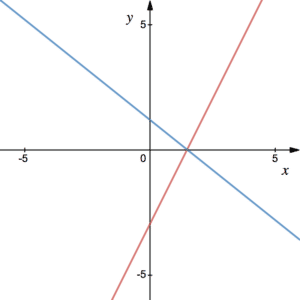# Simultaneous Equations

We can think of simultaneous equations as being two equations in two unknowns, say $x$ and $y$. Note that the word simultaneous means ‘at the same time’. It follows that for the values of $x$ and $y$ found both equations must be true at the same time. Sometimes it is easy to inspect the equations and guess the answers. However, when one of the equations is quadratic this becomes less likely. The answers could be surds, in which case, this is very difficult to guess.

Note that a question may ask you to solve simultaneous equations explicitly. In others, it will be implied and you must deduce that it is simultaneous equations to solve. For example, the question could ask you  to find out which points two curves have in common. See Example 2. (What if there are 3 equations?)

## Methods for Solving Simultaneous Equations

There are three methods for solving simultaneous equations:

1. Elimination– this is where you multiply both equations through by different coefficient in order to eliminate one of the unknowns. This page will focus on substitution since it works for more complicated simultaneous equations. For example, when one of the equations is a quadratic. Click here to see an example using elimination.
2. Substitution – one of the equations can be quadratic, in which case, substitution is the method to use. You will need to know how to solve quadratics. Make $x$ or $y$ the subject of one of the equations, then substitute into the other. See the Worked Example and Example 1.
3. Graphical method – we can interpret the solution of simultaneous equations as the intersection of their graphs. This plot shows the graphs of $y=2x-3$ in red and $4x+5y=6$ in blue. Their intersection lies on the x-axis and has coordinates (1.5,0). This is the solution when solving simultaneously. Also see Example 2.## Simultaneous Equations Worked Example

Solve the simultaneous equations

$x^2+y^2=10$

and

$x+2y=5$.

This example requires solution via substitution, i.e. make either $x$ or $y$ the subject of one equation and insert it into the other. The obvious choice would be to make $x$ the subject of the second equation – it is the quickest, least complicated choice. The second equation tells us that $x=5-2y$. We can insert this into the first equation: $(5-2y)^2+y^2=10$. By multiplying out the brackets and simplifying we see that this is a quadratic equation in $y$:

$(5-2y)^2+y^2=10$

Write out the brackets: $(5-2y)(5-2y)+y^2=10$

Expand the brackets: $25-10y-10y+4y^2+y^2=10$

Simplify: $5y^2-20y+15=0$

Divide both by sides by 5: $y^2-4y+3=0$

Factorise: $(y-3)(y-1)=0$

This tells us that y has to be either 3 or 1. If $y=3$, then $x=5-2\times 3=-1$ (from the second equation rearranged) and if $y=1$ then $x=5-2\times 1=3$.

We obtain the solutions $(x_1,y_1)=(-1,3)$ and $(x_2,y_2)=(3,1)$.

## Examples

Solve the simultaneous equations:

$y=x-4$

$2x^2-xy=8$

Sketch the graphs of $x^2+y^2=10$ and $x+2y=5$ on the same plot. Determine the coordinates of the intersection points.